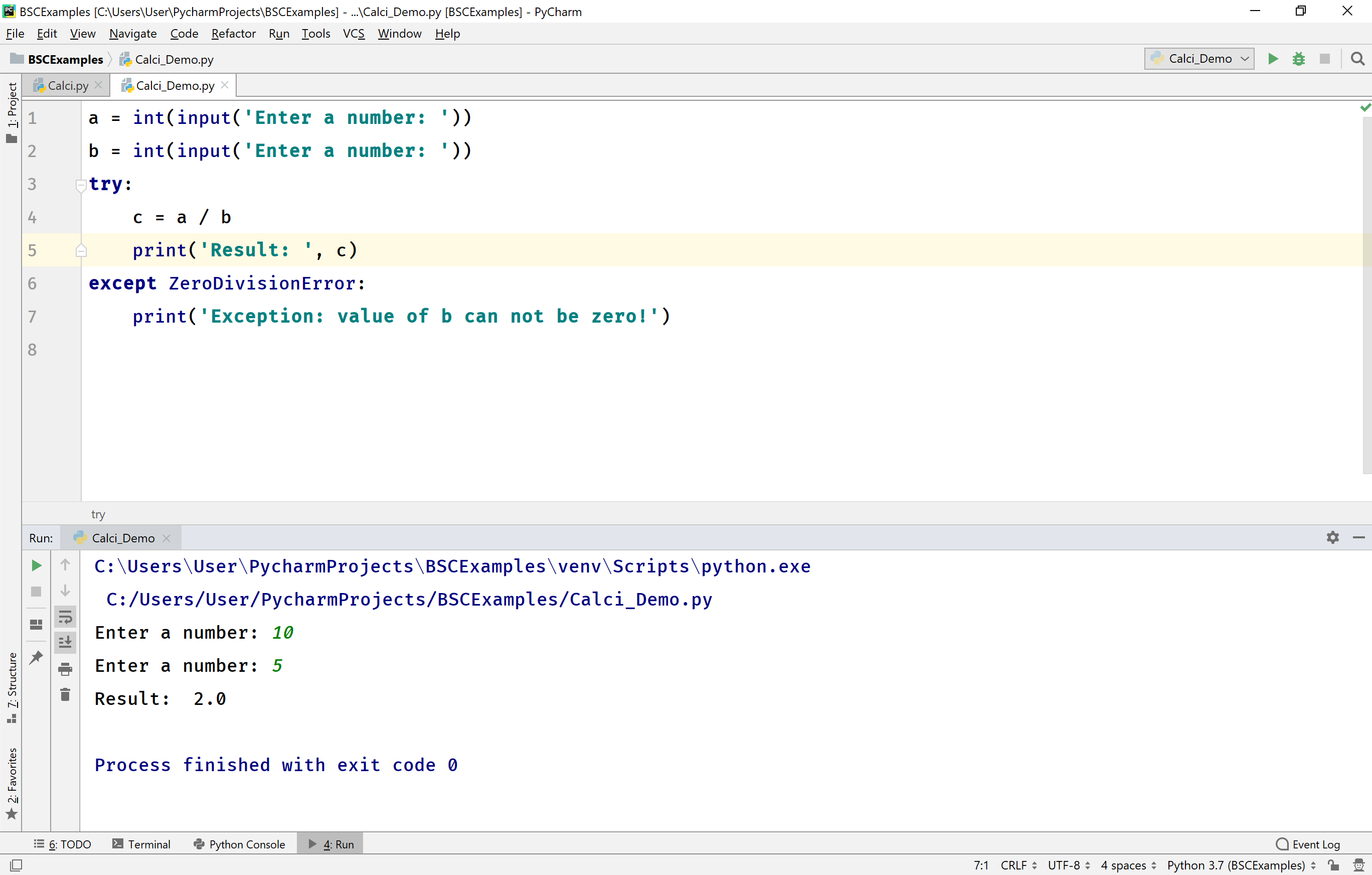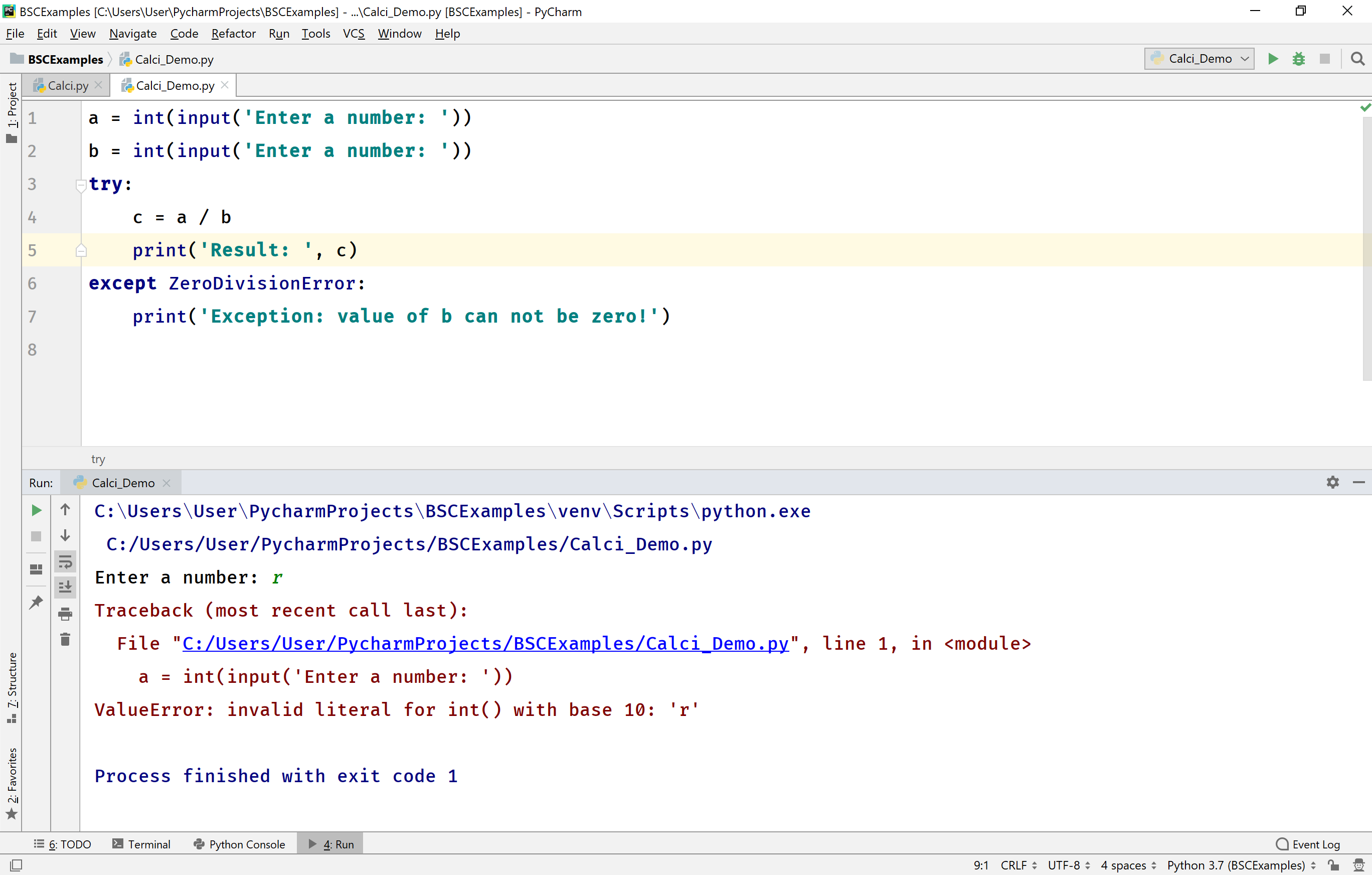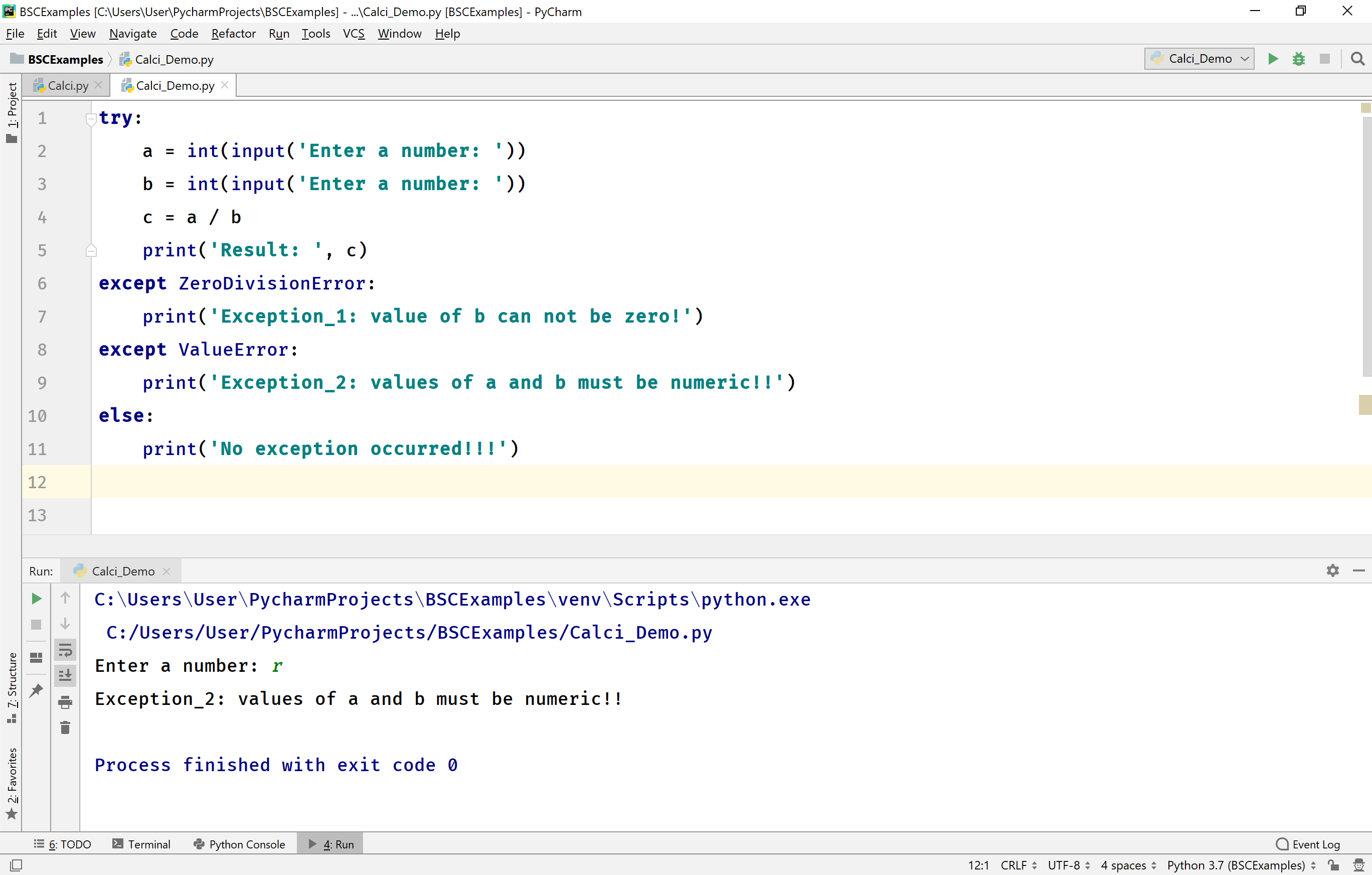The perfect place for easy learning...

×

# Python Exception Handling

In Python, the exception is an abnormal situation during the execution. In general, when a Python program encounters a situation that it cannot cope with, it raises an exception. When an exception has occurred, it must either handle the exception otherwise it terminates the execution.

The Python programming language provides three keywords to handle the exceptions.

• try
• except
• finally

# try

The try keyword is used to define a block of code which may cause an exception.

# except

The except keyword is used to define a block of code which handles the exception.

# finally

The finally keyword is used to define a block of code which is to execute, regardless of an exception occurrence.

The Python allows us to use an optional else keyword that can be used with try block. The else is used to define a block of code to be executed if no exception were raised.

The following is the general syntax of exception handling in the Python.

Syntax
``````try:
# the code that may raise an exception
except:
# the code that handles an exception
else:
# the code that to be executed if no exception were raised
finally:
# the code that must be executed
``````

Let's look at the following example code. The code generates an excetion, because of undefined symbol.

Example
``````try:
a = a * 10
print(a)
except:
print('Exception: Please do define variable a before it is used!')
else:
print('Everything is going smooth!!')
finally:
print('This is executed compulsory!!!')
``````

When we run the above example code, it produces the following output.# Catching the specific Exception

In Python, the except block may also catch a particular exception. In such a case, it handles only the particularized exception, if any other exception occurs it terminates the execution. Let's look at the following piece of code.

Example
``````a = int(input('Enter a number: '))
b = int(input('Enter a number: '))
try:
c = a / b
print('Result: ', c)
except ZeroDivisionError:
print('Exception: value of b can not be zero!')
``````

When we run the above example code, it produces the following output for the values a = 5 and b = 0.When we run the above example code, it produces the following output for the values a = 10 and b = 5.When we run the above example code, it produces the following output for the values a = r and b = 5. Here, the exception is a ValueError which can not be handled by the above code.# Catching Multiple Exceptions

In Python, the except block may also catch multiple exceptions. In such a case, a single try block has multiple except blocks. When an exception arises, it sent to the first except block if it can handle then rest of the except blocks are ignored otherwise it forwarded to the next except block and so on.
The above exmple code may generate two types of exceptions ZeroDivisionError and ValueError. Let's modify the above code to handle both the exception.

Example
``````try:
a = int(input('Enter a number: '))
b = int(input('Enter a number: '))
c = a / b
print('Result: ', c)
except ZeroDivisionError:
print('Exception_1: value of b can not be zero!')
except ValueError:
print('Exception_2: values of a and b must be numeric!!')
else:
print('No exception occurred!!!')
``````

When we run the above example code, it produces the following output for the values a = r and b = 5.